## Based on the above information answer the following questions: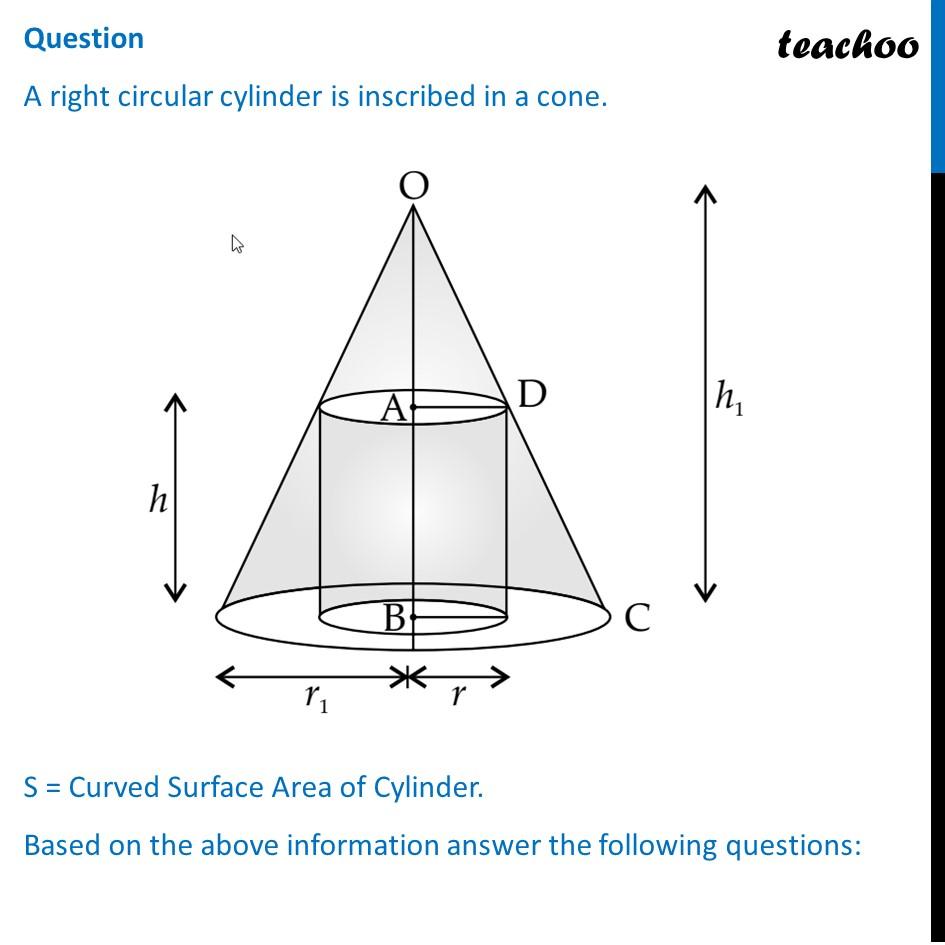## (D) (h + h 1 )/h 1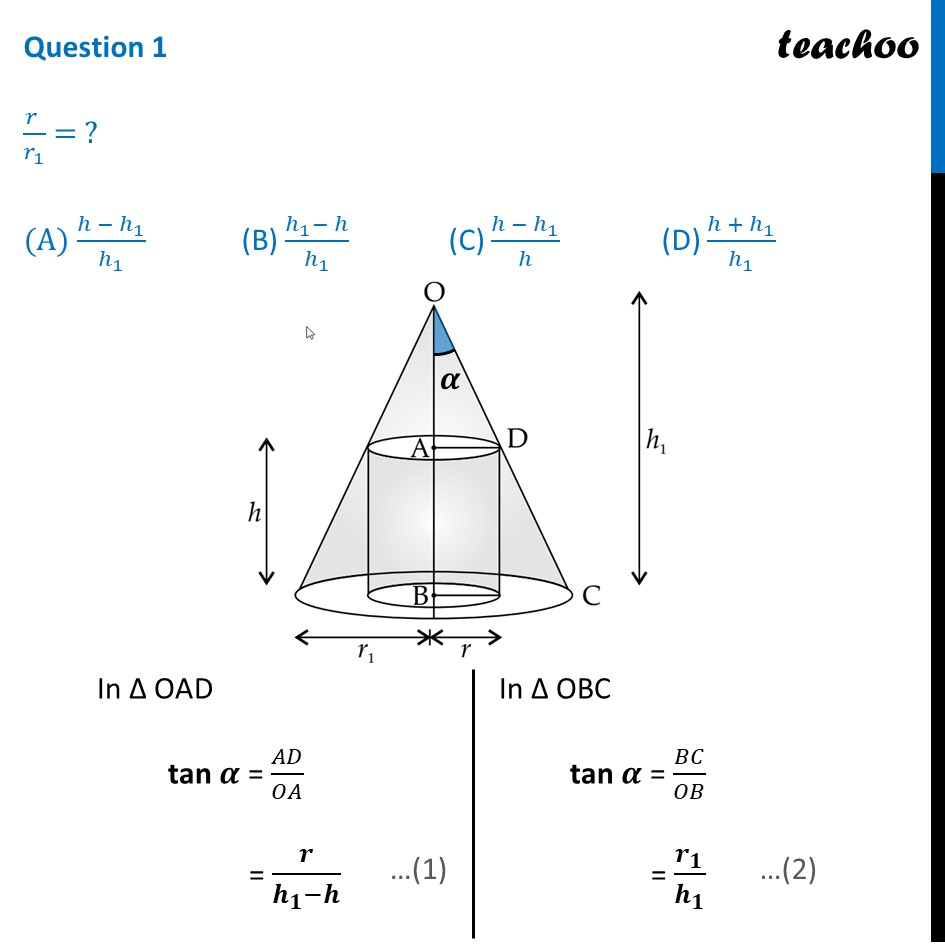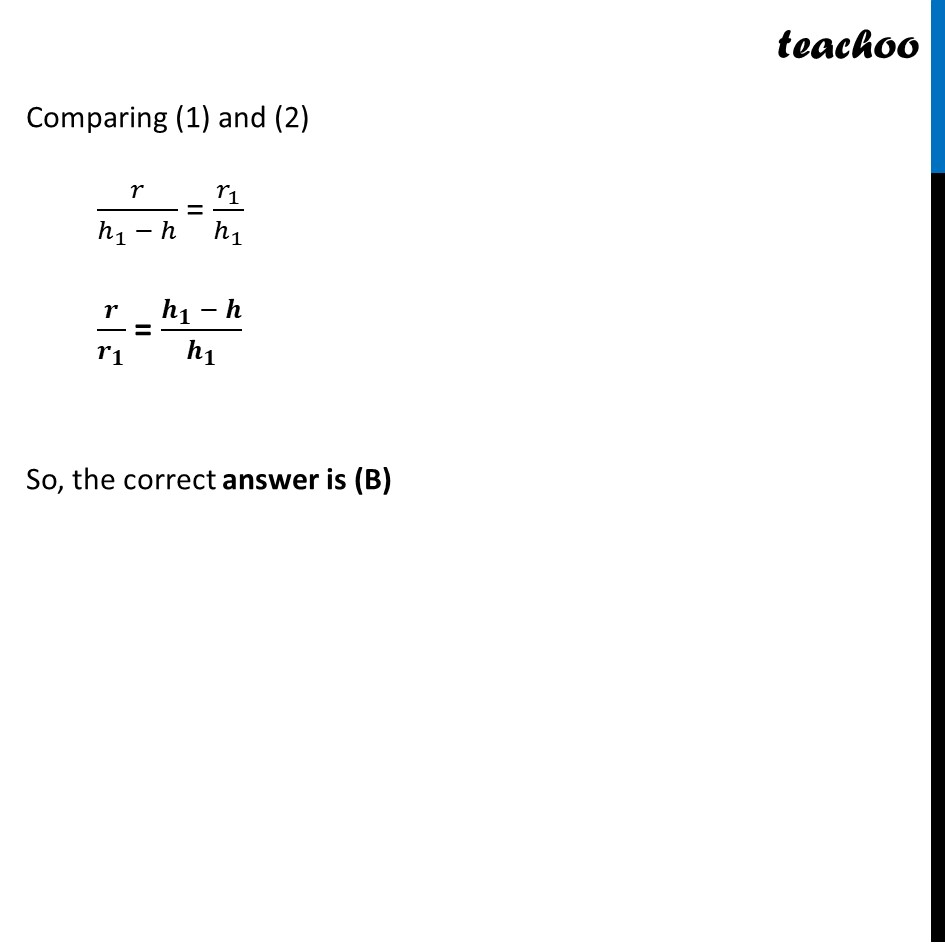## (D) (2πr 1 )/h 1 (h 1 + h)h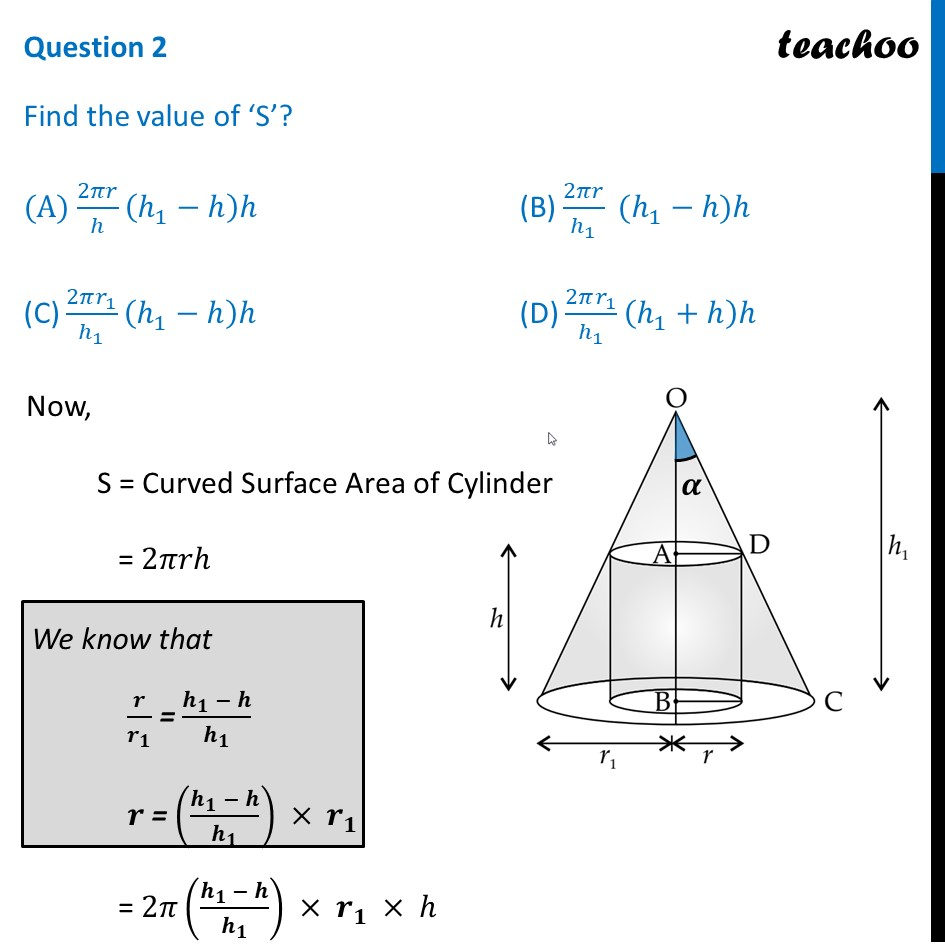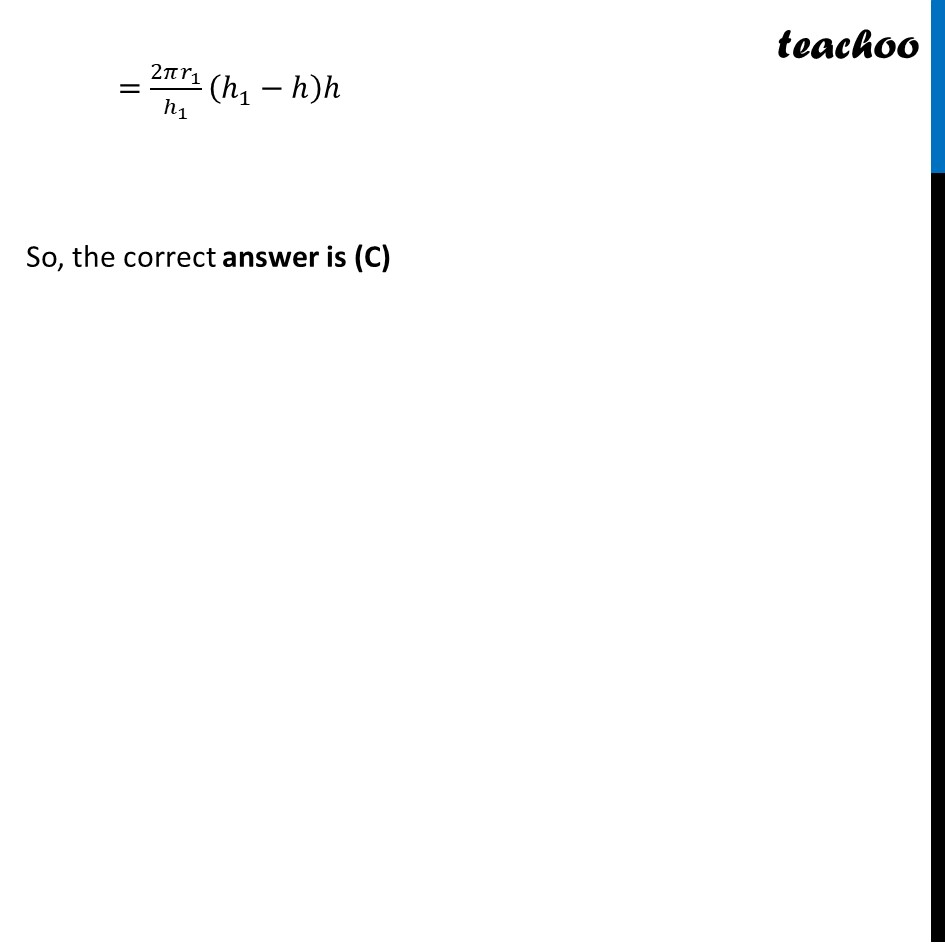## (D) 2πr1/h 1 (h 1 - 2h)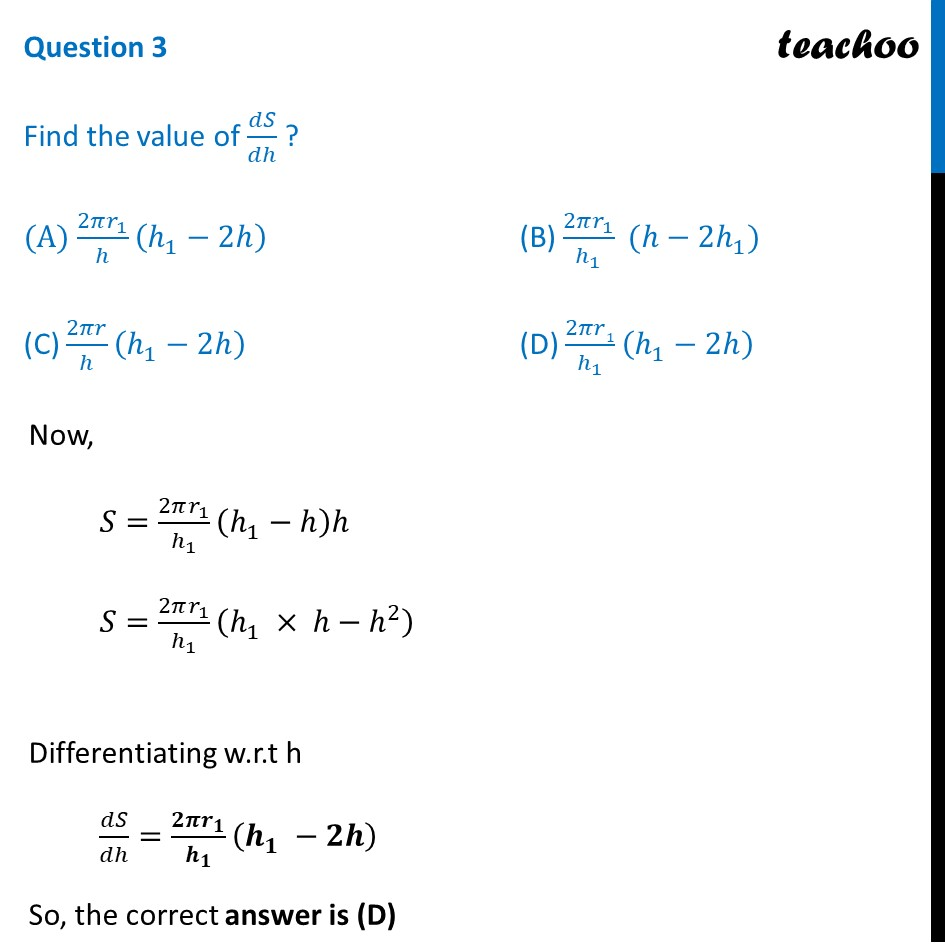## (D) (4πr 1 )/h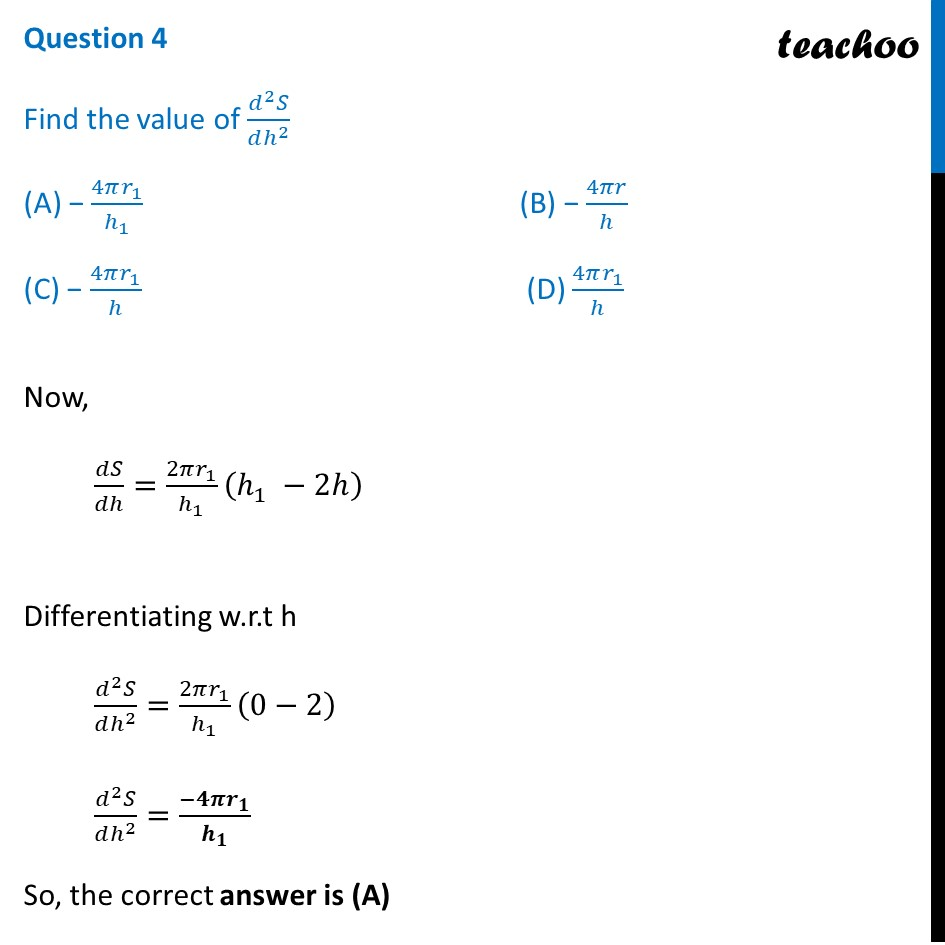## (D) r 1 /2 = r/3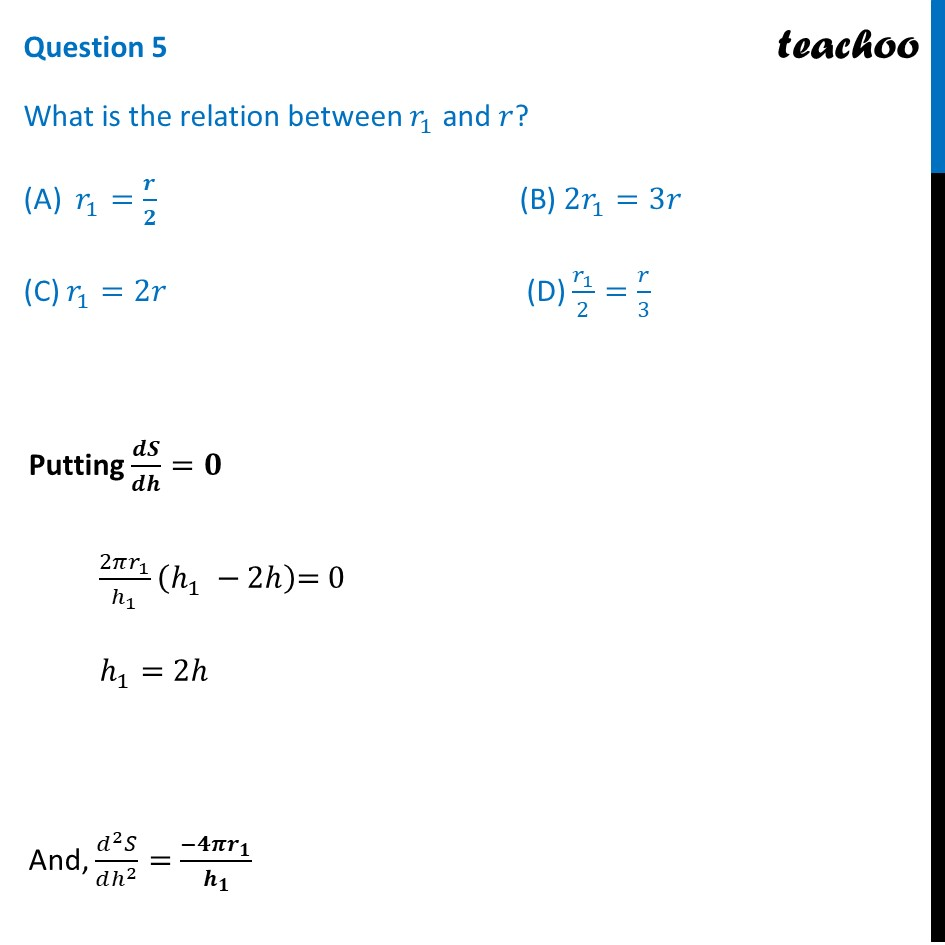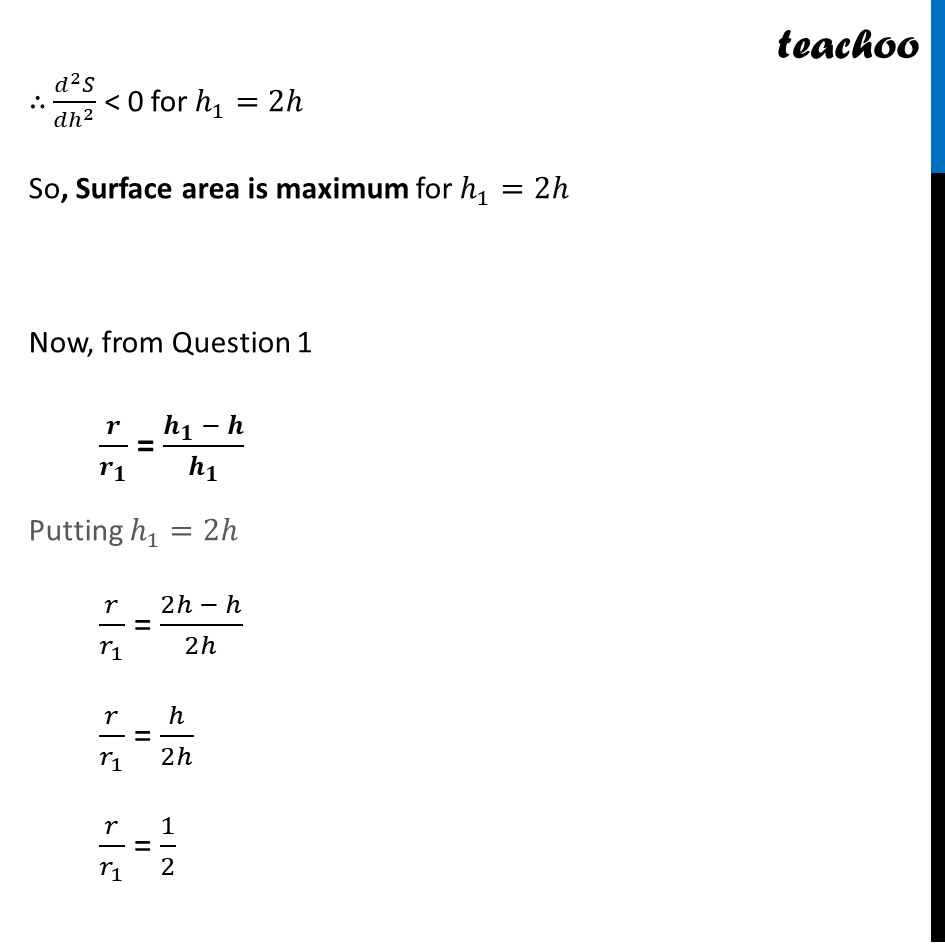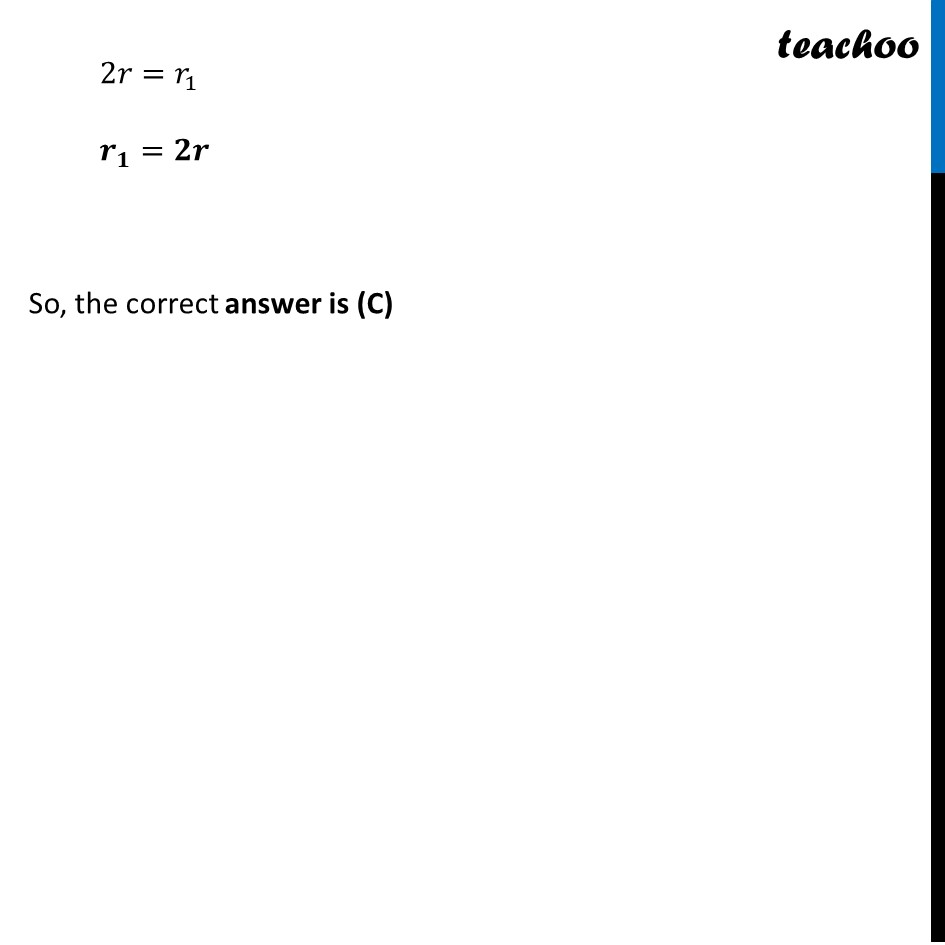1. Chapter 6 Class 12 Application of Derivatives (Term 1)
2. Serial order wise
3. Case Based Questions (MCQ)

Transcript

Question A right circular cylinder is inscribed in a cone. S = Curved Surface Area of Cylinder. Based on the above information answer the following questions: Question 1 (𝑟 )/𝑟1 = ? (A) (ℎ −ℎ1)/ℎ1 (B) (ℎ1−ℎ)/ℎ1 (C) (ℎ −ℎ1)/ℎ (D) (ℎ + ℎ1)/ℎ1 In Δ OAD tan 𝜶 = 𝐴𝐷/𝑂𝐴 = 𝒓/(𝒉_𝟏−𝒉) In Δ OBC tan 𝜶 = 𝐵𝐶/𝑂𝐵 = 𝒓_𝟏/𝒉_𝟏 Comparing (1) and (2) 𝑟/(ℎ_1 − ℎ) = 𝑟_1/ℎ_1 𝒓/𝒓_𝟏 = (𝒉_𝟏 − 𝒉)/𝒉_𝟏 So, the correct answer is (B) Question 2 Find the value of ‘S’? (A) 2𝜋𝑟/ℎ (ℎ_1−ℎ)ℎ (B) 2𝜋𝑟/ℎ_1 (ℎ_1−ℎ)ℎ (C) (2𝜋𝑟_1)/ℎ_1 (ℎ_1−ℎ)ℎ (D) (2𝜋𝑟_1)/ℎ_1 (ℎ_1+ℎ)ℎ Now, S = Curved Surface Area of Cylinder = 2𝜋𝑟ℎ We know that 𝒓/𝒓_𝟏 = (𝒉_𝟏 − 𝒉)/𝒉_𝟏 𝒓 = ((𝒉_𝟏 − 𝒉)/𝒉_𝟏 ) × 𝒓_𝟏 = 2𝜋((𝒉_𝟏 − 𝒉)/𝒉_𝟏 ) × 𝒓_𝟏 × ℎ =(2𝜋𝑟_1)/ℎ_1 (ℎ_1−ℎ)ℎ So, the correct answer is (C) Question 3 Find the value of 𝑑𝑆/𝑑ℎ ? (A) (2𝜋𝑟_1)/ℎ (ℎ_1−2ℎ) (B) (2𝜋𝑟_1)/ℎ_1 (ℎ−2ℎ_1) (C) 2𝜋𝑟/ℎ (ℎ_1−2ℎ) (D) 2𝜋𝑟1/ℎ_1 (ℎ_1−2ℎ) Now, 𝑆=(2𝜋𝑟_1)/ℎ_1 (ℎ_1−ℎ)ℎ 𝑆=(2𝜋𝑟_1)/ℎ_1 (ℎ_1 × ℎ−ℎ^2 ) Differentiating w.r.t h 𝑑𝑆/𝑑ℎ=(𝟐𝝅𝒓_𝟏)/𝒉_𝟏 (𝒉_𝟏 −𝟐𝒉) So, the correct answer is (D) Question 4 Find the value of (𝑑^2 𝑆)/(𝑑ℎ^2 ) (A) − (4𝜋𝑟_1)/ℎ_1 (B) − 4𝜋𝑟/ℎ (C) − (4𝜋𝑟_1)/ℎ (D) (4𝜋𝑟_1)/ℎ Now, 𝑑𝑆/𝑑ℎ=(2𝜋𝑟_1)/ℎ_1 (ℎ_1 −2ℎ)" " Differentiating w.r.t h (𝑑^2 𝑆)/(𝑑ℎ^2 )=(2𝜋𝑟_1)/ℎ_1 (0−2) (𝑑^2 𝑆)/(𝑑ℎ^2 )=(−𝟒𝝅𝒓_𝟏)/𝒉_𝟏 So, the correct answer is (A) Question 5 What is the relation between 𝑟_1 and 𝑟? (A) 𝑟_1=𝒓/𝟐 (B) 2𝑟_1=3𝑟 (C) 𝑟_1=2𝑟 (D) 𝑟_1/2=𝑟/3 Putting 𝒅𝑺/𝒅𝒉=𝟎 (2𝜋𝑟_1)/ℎ_1 (ℎ_1 −2ℎ)"= 0 " ℎ_1=2ℎ And, (𝑑^2 𝑆)/(𝑑ℎ^2 )=(−𝟒𝝅𝒓_𝟏)/𝒉_𝟏 ∴ (𝑑^2 𝑆)/(𝑑ℎ^2 ) < 0 for ℎ_1=2ℎ So, Surface area is maximum for ℎ_1=2ℎ Now, from Question 1 𝒓/𝒓_𝟏 = (𝒉_𝟏 − 𝒉)/𝒉_𝟏 Putting ℎ_1=2ℎ 𝑟/𝑟_1 = (2ℎ − ℎ)/2ℎ 𝑟/𝑟_1 = ℎ/2ℎ 𝑟/𝑟_1 = 1/2 2𝑟=𝑟_1 𝒓_𝟏=𝟐𝒓 So, the correct answer is (C)